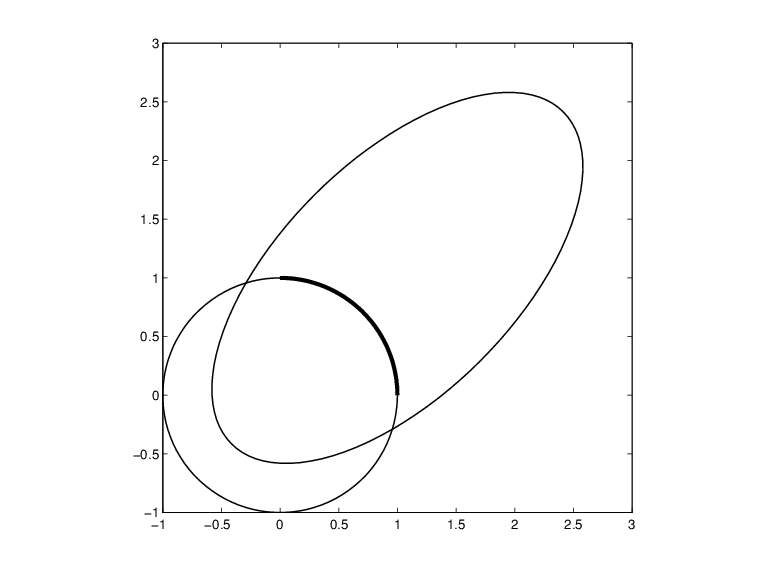## Constructing and Working with Rational Splines

### Rational Spline Example: Circle

For example,

```circle = rsmak('circle'); ```

provides a rational spline whose values on its basic interval trace out the unit circle, i.e., the circle of radius 1 with center at the origin, as the command

```fnplt(circle), axis square ```

readily shows; the resulting output is the circle in the figure A Circle and an Ellipse, Both Given by a Rational Spline.

It is easy to manipulate this circle to obtain related shapes. For example, the next commands stretch the circle into an ellipse, rotate the ellipse 45 degrees, and translate it by (1,1), and then plot it on top of the circle.

```ellipse = fncmb(circle,[2 0;0 1]); s45 = 1/sqrt(2); rtellipse = fncmb(fncmb(ellipse, [s45 -s45;s45 s45]), [1;1] ); hold on, fnplt(rtellipse), hold off ```

As a further example, the "circle" just constructed is put together from four pieces. To highlight the first such piece, use the following commands:

```quarter = fnbrk(fn2fm(circle,'rp'),1); hold on, fnplt(quarter,3), hold off ```

In the first command, `fn2fm` is used to change forms, from one based on the B-form to one based on the ppform, and then `fnbrk` is used to extract the first piece, and this piece is then plotted on top of the circle in A Circle and an Ellipse, Both Given by a Rational Spline, with linewidth `3` to make it stand out.

A Circle and an Ellipse, Both Given by a Rational Spline### Rational Spline Example: Sphere

As a surface example, the command `rsmak('southcap')` provides a 3-vector valued rational bicubic polynomial whose values on the unit square [-1 .. 1]^2 fill out a piece of the unit sphere. Adjoin to it five suitable rotates of it and you get the unit sphere exactly. For illustration, the following commands generate two-thirds of that sphere, as shown in Part of a Sphere Formed by Four Rotates of a Quartic Rational.

```southcap = rsmak('southcap'); fnplt(southcap) xpcap = fncmb(southcap,[0 0 -1;0 1 0;1 0 0]); ypcap = fncmb(xpcap,[0 -1 0; 1 0 0; 0 0 1]); northcap = fncmb(southcap,-1); hold on, fnplt(xpcap), fnplt(ypcap), fnplt(northcap) axis equal, shading interp, view(-115,10), axis off, hold off ```

Part of a Sphere Formed by Four Rotates of a Quartic Rational### Functions for Working With Rational Splines

Having chosen to represent the rational spline r = s/w in this way by the ordinary spline R=[s;w] makes it is easy to apply to a rational spline all the `fn...` commands in the Curve Fitting Toolbox™ spline functions, with the following exceptions. The integral of a rational spline need not be a rational spline, hence there is no way to extend `fnint` to rational splines. The derivative of a rational spline is again a rational spline but one of roughly twice the order. For that reason, `fnder` and `fndir` will not touch rational splines. Instead, there is the command `fntlr` for computing the value at a given `x` of all derivatives up to a given order of a given function. If that function is rational, the needed calculation is based on the considerations given in the preceding paragraph.

The command `r = rsmak(shape)` provides rational splines in rBform that describe exactly certain standard geometric shapes , like `'circle'`, `'arc'`, `'cylinder'`, `'sphere'`, `'cone'`, `'torus'`. The command `fncmb(r,trans)` can be used to apply standard transformations to the resulting shape. For example, if `trans` is a column-vector of the right length, the shape would be translated by that vector while, if `trans` is a suitable matrix like a rotation, the shape would be transformed by that matrix.

The command `r = rscvn(p)` constructs the quadratic rBform of a tangent-continuous curve made up of circular arcs and passing through the given sequence, `p`, of points in the plane.

A special rational spline form, called a NURBS, has become a standard tool in CAGD. A NURBS is, by definition, any rational spline for which both s and w are in the same B-form, with each coefficient for s containing explicitly the corresponding coefficient for w as a factor:

`$\begin{array}{cc}s=\sum _{i}{B}_{i}v\left(i\right)a\left(:,i\right),& w=\sum _{i}{B}_{i}v\left(i\right)\end{array}$`

The normalized coefficients a(:,i) for the numerator spline are more readily used as control points than the unnormalized coefficients v(i)a(:,i) used in the rBform. Nevertheless, this toolbox provides no special NURBS form, but only the more general rational spline, but in both B-form (called `rBform` internally) and in ppform (called `rpform` internally).

The rational spline `circle` used earlier is put together in `rsmak` by code like the following.

```x = [1 1 0 -1 -1 -1 0 1 1]; y = [0 1 1 1 0 -1 -1 -1 0]; s45 = 1/sqrt(2); w =[1 s45 1 s45 1 s45 1 s45 1]; circle = rsmak(augknt(0:4,3,2), [w.*x;w.*y;w]); ```

Note the appearance of the denominator spline as the last component. Also note how the coefficients of the denominator spline appear here explicitly as factors of the corresponding coefficients of the numerator spline. The normalized coefficient sequence `[x;y]` is very simple; it consists of the vertices and midpoints, in proper order, of the "unit square". The resulting control polygon is tangent to the circle at the places where the four quadratic pieces that form the circle abut.

For a thorough discussion of NURBS, see [G. Farin, NURBS, 2nd ed., AKPeters Ltd, 1999] or [Les Piegl and Wayne Tiller, The NURBS Book, 2nd ed., Springer-Verlag, 1997].